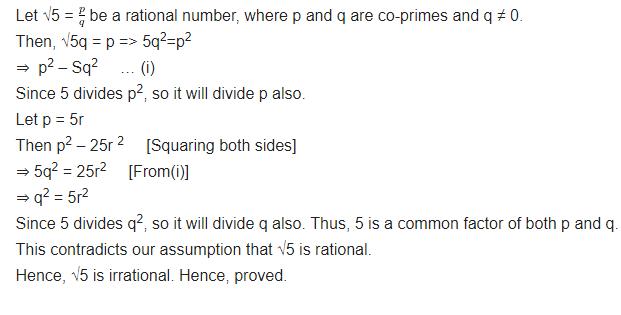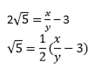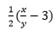• +91 9971497814
• info@interviewmaterial.com

# Real Numbers Ex-1.3 Interview Questions Answers

### Related Subjects

Question 1 : Prove that √is irrational.

Answer 1 :Question 2 :

Prove that 3 + 2√5 + is irrational.

Solutions:

Letus 3 + 25 is rational.

Then we can find co-prime x and y (y ≠ 0)

Then 3+ 2√5 = x/y

Rearranging, we get,Since, x and y are integers, thus,is a rational number.

Therefore, 5 is also a rationalnumber. But this contradicts the fact that 5 is irrational.

So, we conclude that 3 + 25 isirrational.

Question 3 :

Prove that the following are irrationals:-

(i) 1/√2

(ii) 7√5

(iii) 6 + 2

Q (i)     1/√2

Solutions:

(i) 1/2

Let us   1/√2 is rational.

Then we can find co-prime x and y (y ≠ 0)

Then1/√2 = x/y

Rearranging, we get,

√2 = y/x

Since, x and y are integers, thus, √2 is arational number, which contradicts the fact that √2 is irrational.

Hence, we can conclude that 1/√2 isirrational.

Q (ii)    7√5

Solutions:

(ii) 75

Let us 7√5 is a rational number.

Then we can find co-prime a and b (b ≠ 0)

Then  7√5= x/y

Rearranging, we get,

√5 = x/7y

Since, x and y are integers, thus, √5 is arational number, which contradicts the fact that √5 is irrational.

Hence, we can conclude that 7√5 is irrational.

Q (iii)   6 + 2

Solutions:

(iii) 6+2

Let us 6 +√2 is a rational number.

Then we can find co-primes x and y (y ≠ 0)

Then   6 +√2 = x/y

Rearranging, we get,

√2 = (x/y) – 6

Since, x and y are integers, thus (x/y) – 6 isa rational number and therefore, √2 is rational. This contradicts the fact that√2 is an irrational number.

Hence, we can conclude that 6 +√2 is irrational.

Todays Deals### Real Numbers Ex-1.3 Contributorskrishan

Name:
Email:

# Latest News# 9000 interview questions in different categories